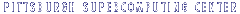|   Search  |   Site Map  |   Help  |   Contacts# The IEEE standard for floating point arithmetic

The IEEE (Institute of Electrical and Electronics Engineers) has produced a standard for floating point arithmetic. This standard specifies how single precision (32 bit) and double precision (64 bit) floating point numbers are to be represented, as well as how arithmetic should be carried out on them.

At the PSC, the Ultrix front-ends use the IEEE format. The VMS front-ends and Cray C90 systems have their own vendor-specific formats for data.

Because many of our users may have occasion to transfer unformatted or "binary" data between an IEEE machine and the Cray or the VAX/VMS, it is worth noting the details of this format for comparison with the Cray and VAX representations. The differences in the formats also affect the accuracy of floating point computations.

## Summary:

### Single Precision

The IEEE single precision floating point standard representation requires a 32 bit word, which may be represented as numbered from 0 to 31, left to right. The first bit is the sign bit, S, the next eight bits are the exponent bits, 'E', and the final 23 bits are the fraction 'F':

```  S EEEEEEEE FFFFFFFFFFFFFFFFFFFFFFF
0 1      8 9                    31
```

The value V represented by the word may be determined as follows:

• If E=255 and F is nonzero, then V=NaN ("Not a number")
• If E=255 and F is zero and S is 1, then V=-Infinity
• If E=255 and F is zero and S is 0, then V=Infinity
• If 0<E<255 then V=(-1)**S * 2 ** (E-127) * (1.F) where "1.F" is intended to represent the binary number created by prefixing F with an implicit leading 1 and a binary point.
• If E=0 and F is nonzero, then V=(-1)**S * 2 ** (-126) * (0.F) These are "unnormalized" values.
• If E=0 and F is zero and S is 1, then V=-0
• If E=0 and F is zero and S is 0, then V=0

In particular,

```  0 00000000 00000000000000000000000 = 0
1 00000000 00000000000000000000000 = -0

0 11111111 00000000000000000000000 = Infinity
1 11111111 00000000000000000000000 = -Infinity

0 11111111 00000100000000000000000 = NaN
1 11111111 00100010001001010101010 = NaN

0 10000000 00000000000000000000000 = +1 * 2**(128-127) * 1.0 = 2
0 10000001 10100000000000000000000 = +1 * 2**(129-127) * 1.101 = 6.5
1 10000001 10100000000000000000000 = -1 * 2**(129-127) * 1.101 = -6.5

0 00000001 00000000000000000000000 = +1 * 2**(1-127) * 1.0 = 2**(-126)
0 00000000 10000000000000000000000 = +1 * 2**(-126) * 0.1 = 2**(-127)
0 00000000 00000000000000000000001 = +1 * 2**(-126) *
0.00000000000000000000001 =
2**(-149)  (Smallest positive value)
```

### Double Precision

The IEEE double precision floating point standard representation requires a 64 bit word, which may be represented as numbered from 0 to 63, left to right. The first bit is the sign bit, S, the next eleven bits are the exponent bits, 'E', and the final 52 bits are the fraction 'F':

```  S EEEEEEEEEEE FFFFFFFFFFFFFFFFFFFFFFFFFFFFFFFFFFFFFFFFFFFFFFFFFFFF
0 1        11 12                                                63
```

The value V represented by the word may be determined as follows:

• If E=2047 and F is nonzero, then V=NaN ("Not a number")
• If E=2047 and F is zero and S is 1, then V=-Infinity
• If E=2047 and F is zero and S is 0, then V=Infinity
• If 0<E<2047 then V=(-1)**S * 2 ** (E-1023) * (1.F) where "1.F" is intended to represent the binary number created by prefixing F with an implicit leading 1 and a binary point.
• If E=0 and F is nonzero, then V=(-1)**S * 2 ** (-1022) * (0.F) These are "unnormalized" values.
• If E=0 and F is zero and S is 1, then V=-0
• If E=0 and F is zero and S is 0, then V=0

## Reference:

ANSI/IEEE Standard 754-1985,
Standard for Binary Floating Point Arithmetic|   Software  |   Feedback  |   Home